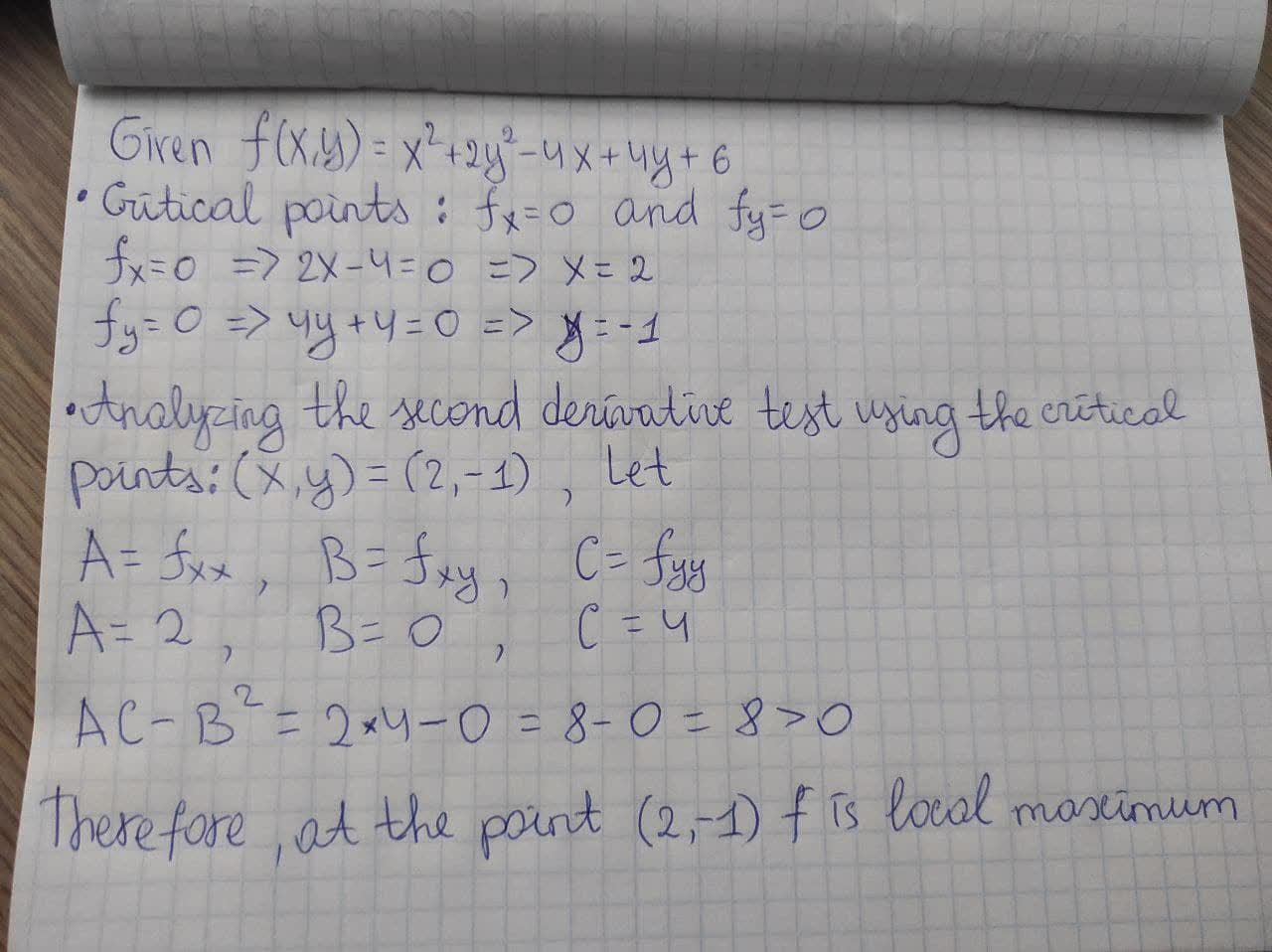# Use the Second Derivative Test to classify the critical points of f(x, y) = x^2 + 2y^2 - 4x + 4y + 6.Ayaana Buck 2021-02-02 Answered
Use the Second Derivative Test to classify the critical points of $f\left(x,y\right)={x}^{2}+2{y}^{2}-4x+4y+6.$
You can still ask an expert for help

• Questions are typically answered in as fast as 30 minutes

Solve your problem for the price of one coffee

• Math expert for every subject
• Pay only if we can solve ittafzijdeq

To find the critical points : ${f}_{x}=0$ and ${f}_{y}=0$
${f}_{x}=0⇒2x-4=0⇒x=2$
and ${f}_{y}=0⇒4y+4=0⇒y=-1$
Analyzing the second derivative test using the critical point: (x,y)=(2, -1) Let
$A={f}_{xx},B={f}_{xy},$ and $C={f}_{yy}$
$A={f}_{xx}=2,B={f}_{xy}=0,$ and $C={f}_{yy}=4$
$AC-{B}^{2}=8-0=8>0$
Therefore, at the point (2, -1) f is local maximum.

###### Not exactly what you’re looking for?Jeffrey Jordon

Photo explanation